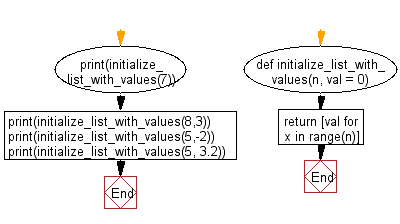﻿ Python: Initialize and fills a list with the specified value - w3resource# Python: Initialize and fills a list with the specified value

## Python List: Exercise - 251 with Solution

Write a Python program to initialize and fills a list with the specified value.

• Use a list comprehension and range() to generate a list of length equal to n, filled with the desired values.
• Omit val to use the default value of 0.

Sample Solution:

Python Code:

``````def initialize_list_with_values(n, val = 0):
return [val for x in range(n)]
print(initialize_list_with_values(7))
print(initialize_list_with_values(8,3))
print(initialize_list_with_values(5,-2))
print(initialize_list_with_values(5, 3.2))
```
```

Sample Output:

```[0, 0, 0, 0, 0, 0, 0]
[3, 3, 3, 3, 3, 3, 3, 3]
[-2, -2, -2, -2, -2]
[3.2, 3.2, 3.2, 3.2, 3.2]
```

Flowchart:## Visualize Python code execution:

The following tool visualize what the computer is doing step-by-step as it executes the said program:

Python Code Editor:

Have another way to solve this solution? Contribute your code (and comments) through Disqus.

What is the difficulty level of this exercise?

Test your Python skills with w3resource's quiz

﻿

## Python: Tips of the Day

Floor Division:

When we speak of division we normally mean (/) float division operator, this will give a precise result in float format with decimals.

For a rounded integer result there is (//) floor division operator in Python. Floor division will only give integer results that are round numbers.

```print(1000 // 300)
print(1000 / 300)```

Output:

```3
3.3333333333333335```